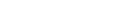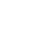# Visualizations

Visualizations are powerful aggregations that are run across your data to produce insights that are easy to understand and monitor.

With Visualizations, teams can create and obtain data stats, group fields and observe methods in running deployments.

This page will introduce you to the visualizations supported by Axiom and some tips on how best to use them.

## `count`

The `count` visualization will count all matching events and produces a timeseries chart.

`none`

#### Group-By Behaviour

Visualization will produce a separate result for each group plotted on a timeseries chart.## `distinct`

The `distinct` visualization counts each distinct occurrance of the distinct field inside the dataset and produce a timeseries chart.

#### Arguments

• `field: any` - a field to aggregate

#### Group-By Behaviour

Visualization will produce a seperate result for each group plotted on a timeseries chart.## `avg`

The `avg` visualization averages the values of the field inside the dataset and produces a timeseries chart

#### Arguments

• `field: number` - a number field

#### Group-By Behaviour

Visualization will produce a seperate result for each group plotted on a timeseries chart.## `max`

The `max` visualization finds the maximum value of the field inside the dataset and produces a timeseries chart.

#### Arguments

• `field: number` - a number field

#### Group-By Behaviour

Visualization will produce a seperate result for each group plotted on a timeseries chart.## `min`

The `min` visualization finds the minimum value of the field inside the dataset and produces a timeseries chart.

#### Arguments

• `field: number` - a number field

#### Group-By Behaviour

Visualization will produce a seperate result for each group plotted on a timeseries chart.## `sum`

The `sum` visualization adds all the values of the field inside the dataset and produces a timeseries chart.

#### Arguments

• `field: number` - a number field

#### Group-By Behaviour

Visualization will produce a seperate result for each group plotted on a timeseries chart.## `percentiles`

The `percentiles` visualization calculates the requested percentiles of the field in the dataset and produces a timeseries chart.

#### Arguments

• `field: number` - a number field
• `percentiles: number [, ...]` - one or more percentiles (a float between 0 and 100)
• e.g. `percentiles(request_size, 95, 99, 99.9)`

#### Group-By Behaviour

Visualization will produce a seperate result for each group plotted on a horizonal bar chart, allowing for visual comparison across the groups.## `histogram`

The `histogram` visualization buckets the field into a distribution of N buckets, returning a timeseries heatmap chart.

#### Arguments

• `field: number` - a number field
• `nBuckets`: number of buckets to return
• e.g. `histogram(request_size, 15)`

#### Group-By Behaviour

Visualization will produce a seperate result for each group plotted on a timeseries histogram. Hovering over a group in the totals table will show only the results for that group in the histogram.## `topk`

The `topk` visualization calculates the top values for a field in a dataset. Where `k` can be 10, 20 or any number you specify.

#### Arguments

• `field: string` - a string field
• `nResults`: number of results to return
• e.g. `topk(method, 10)`

#### Group-By Behaviour

Visualization will produce a seperate result for each group plotted on a timeseries chart.Unable to retrieve cloud status